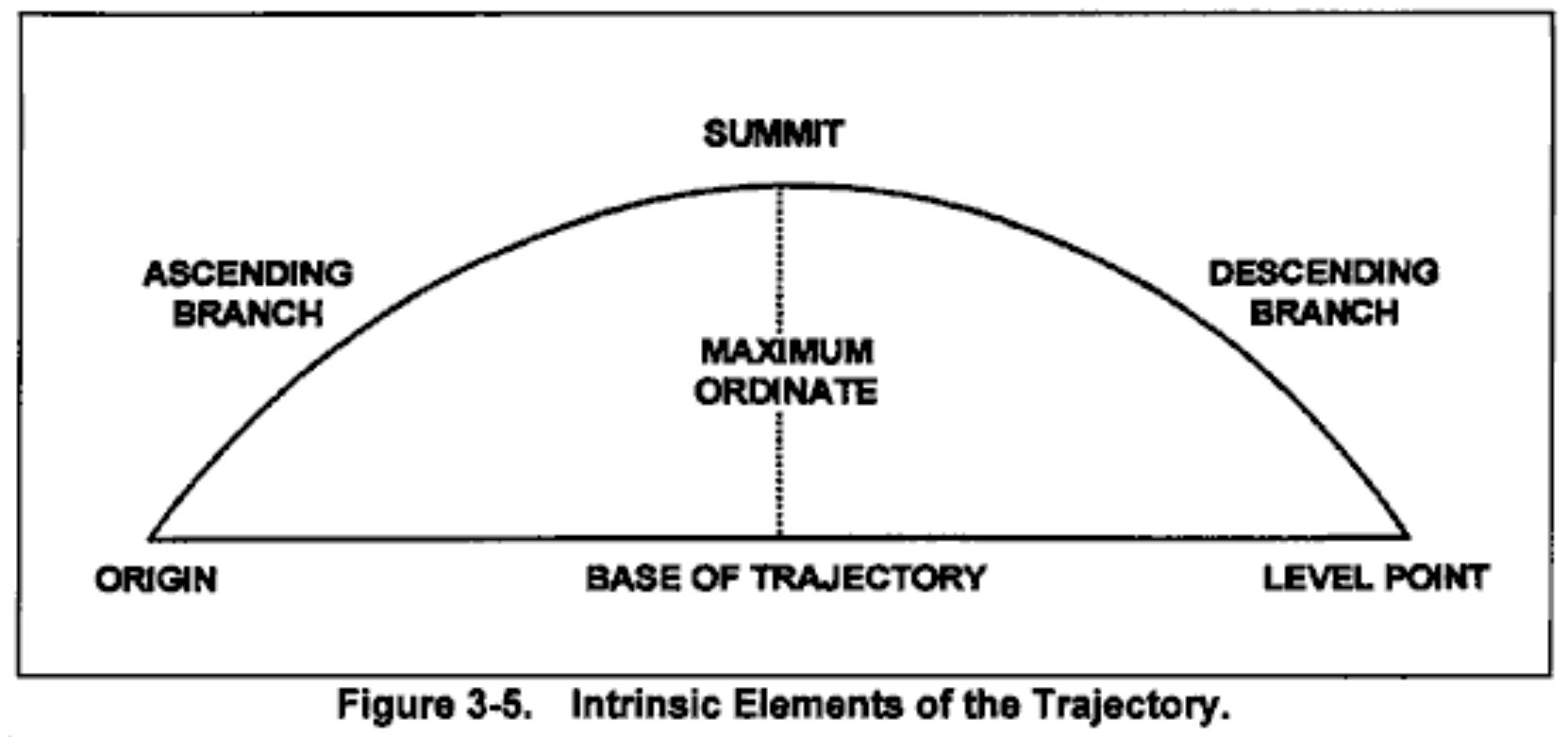# Iterate Trajectory Calculations in Preparation for DPRG Meet

Tags: think, control, and connect
Personhours: 15
Iterate Trajectory Calculations in Preparation for DPRG Meet By Bhanaviya, Mahesh, and Ben

### Task: Improve the Trajectory CalculationsAs mentioned in our earlier posts, one of the biggest control challenges we face in this year's season is identifying a equation to model how the path of a ring launched from our launcher is affected by its angle of launch. 2 weeks ago, we were able to create a starter equation to model this trajectory. However, this time, we want to be able to identify time as not a variable but as a fixed constant and values for RPM, muzzle velocity and rotations per second of a motor. We want to be able to list these values in anticipation of our virtual meeting with the Dallas Personal Robotics this coming week where we will present both our Flywheel Launcher and the calculations we have derived so far. We anticipate that these calculations will be subject to change and our error as we go about the process of identifying how best to put our equations into code and as such, the values in this post are not final in any way.

Our main purpose for modelling this equation is to correct the efficacy of our launcher - primarily what motor it uses and how the motor's PID values need to be fine tuned in order to provide us an ideal launch. In our previous post, we stated that the variables and constants we needed to derive the equations were:

$$\theta$$ - angle of launch

$$hv_0$$ - initial horizontal velocity of launch

$$hv_0$$ - initial horizontal velocity of launch

$$h$$)- height of goal at the third level which we know to be 0.88m

$$d$$) - the horizontal distance between the goal and the robot

$$g$$ (approximately $$9.8 \frac{m}{s^2}$$) - acceleration due to gravity

We initially created two equations - to represent the initial velocity of the robot horizontally and vertically. However, one key component which we originally considered to be manually calculated was time. In order to isolate time as a fixed variable, we needed to find the amount of time in seconds it would take a ring launched from the robot to reach 0 vertical velocity. We knew that for any shot that crosses through the goal with zero vertical speed, the ring needed to have an initial upward velocity such that the acceleration due to gravity brings it to zero vertical velocity at the point it reaches our target height. Regardless of what the horizontal component is, target time in this situation is governed by solely by gravity and vertical distance. Although we had initially modelled our equations to reach the summit in order to make the actual angle of launch more solvable since that would mean that we didn't have to consider "balancing" the initial height of the robot to derive the value of $$\theta$$. However, seeing as the summit is the center of the portion of the trajectory with the least vertical travel for a given span of time or distance, we would not only be able to isolate time but also find $$\theta$$ with the least vertical error. As such, we modelled an equation for time which can be found as shown below.

$\displaylines{t = \sqrt{v_0/(0.5* $$g$$)}}$

In addition to time, we also needed to find the RPM of the motor of the launcher, for which we needed to find the circumference of the launcher, which was 0.48066m. From here, we found the radius to be approximately 0.076m, which we could use for our RPM equation which was RPM = v = \omega r, with r representing 0.076m. To find muzzle velocity, we plan on using our velocity formula as of now but this is likely to change as we continue to inspect our equation. Here are the overall equations we have developed so far now that we know how to look for time at the apex. $\displaylines{d/cos(\theta)(t/2) = hv_0 \\ ((0.88 - 0.41) - 0.5(a)t^2)/sin(t/2)= vv_0, t = \sqrt{v_0/(0.5* $$g$$)}}$

### Next Steps:

Using these equations, we plan to be able to identify angle of launch, RPM and muzzle velocity for a range of distances away from the goal. Mainly, we plan to derive values for a very specific range (likely 2 to 2.5m) to present our calculations as well as our equations to Dallas Personal Robotics Group in the upcoming meeting on Tuesday.

Date | January 24, 2021